# Using ggplot2 package in R Programming

• Last Updated : 09 Nov, 2021

ggplot2 also termed as Grammar of Graphics is a free, opensource, and easy to use visualization package widely used in R. It is the most powerful visualization package written by Hadley Wickham.

It includes several layers on which it is governed. The layers are as follows: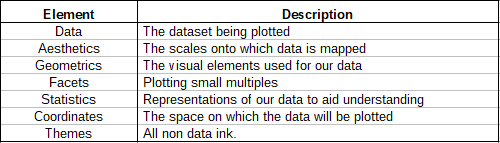#### Building Blocks of layers

Layers with variables of interest are as follows:

• Aesthetics: x-axis, y-axis, color, fill, size, labels, alpha, shape, line width, line type
• Geometrics: point, line, histogram, bar, boxplot
• Facets: Columns, rows
• Statistics: Binning, smoothing, descriptive, intermediate
• Coordinates: Cartesian, fixed, polar, limits

#### The Dataset

mtcars(motor trend car road test) comprise fuel consumption and 10 aspects of automobile design and performance for 32 automobiles and come pre-installed with dplyr package in R.

## Python3

 `# Installing the package``install.packages(``"dplyr"``)` `# Loading package``library(dplyr)` `# Summary of dataset in package``summary(mtcars)`

#### Performing ggplot2 on dataset

We devise visualizations on mtcars dataset which includes 32 car brands and 11 attributes using ggplot2 layers.

## Python3

 `# Installing the package``install.packages(``"ggplot2"``)`` ` `# Loading packages``library(ggplot2)``library(dplyr)`` ` `# Data Layer``ggplot(data ``=` `mtcars)`` ` `# Aesthetic Layer``ggplot(data ``=` `mtcars, aes(x ``=` `hp, y ``=` `mpg, col ``=` `disp))`` ` `# Geometric layer``ggplot(data ``=` `mtcars,``       ``aes(x ``=` `hp, y ``=` `mpg, col ``=` `disp)) ``+` `geom_point()`` ` `# Adding size``ggplot(data ``=` `mtcars,``       ``aes(x ``=` `hp, y ``=` `mpg, size ``=` `disp)) ``+` `geom_point()`` ` `# Adding color and shape``ggplot(data ``=` `mtcars,``       ``aes(x ``=` `hp, y ``=` `mpg, col ``=` `factor(cyl),``                          ``shape ``=` `factor(am))) ``+` `geom_point()`` ` `# Histogram plot``ggplot(data ``=` `mtcars, aes(x ``=` `hp)) ``+``       ``geom_histogram(binwidth ``=` `5``)`` ` `# Facet Layer``p <``-` `ggplot(data ``=` `mtcars,``            ``aes(x ``=` `hp, y ``=` `mpg,``                ``shape ``=` `factor(cyl))) ``+` `geom_point()`` ` `# Separate rows according to transmission type``p ``+` `facet_grid(am ~ .)`` ` `# Separate columns according to cylinders``p ``+` `facet_grid(. ~ cyl)`` ` `# Statistics layer``ggplot(data ``=` `mtcars, aes(x ``=` `hp, y ``=` `mpg)) ``+``                               ``geom_point() ``+``       ``stat_smooth(method ``=` `lm, col ``=` `"red"``)`` ` `# Coordinates layer: Control plot dimensions``ggplot(data ``=` `mtcars, aes(x ``=` `wt, y ``=` `mpg)) ``+``                               ``geom_point() ``+``      ``stat_smooth(method ``=` `lm, col ``=` `"red"``) ``+`` ``scale_y_continuous(``"mpg"``, limits ``=` `c(``2``, ``35``),``                          ``expand ``=` `c(``0``, ``0``)) ``+``  ``scale_x_continuous(``"wt"``, limits ``=` `c(``0``, ``25``),``            ``expand ``=` `c(``0``, ``0``)) ``+` `coord_equal()`` ` `# Add coord_cartesian() to proper zoom in``ggplot(data ``=` `mtcars, aes(x ``=` `wt, y ``=` `hp, col ``=` `am)) ``+``                        ``geom_point() ``+` `geom_smooth() ``+``                        ``coord_cartesian(xlim ``=` `c(``3``, ``6``))`` ` `# Theme layer``ggplot(data ``=` `mtcars, aes(x ``=` `hp, y ``=` `mpg)) ``+``         ``geom_point() ``+` `facet_grid(. ~ cyl) ``+``        ``theme(plot.background ``=` `element_rect(``            ``fill ``=` `"black"``, colour ``=` `"gray"``))`` ` `ggplot(data ``=` `mtcars, aes(x ``=` `hp, y ``=` `mpg)) ``+``        ``geom_point() ``+` `facet_grid(am ~ cyl) ``+``        ``theme_gray()`

Outputs:
Geometric layer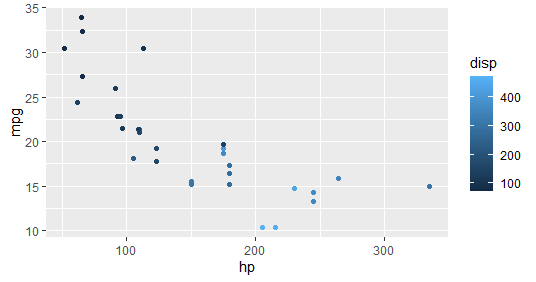• Geometric layer – Adding Size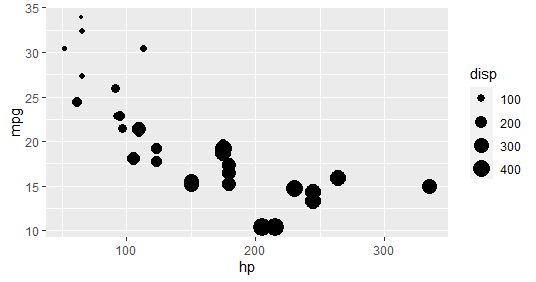• Geometric layer – Adding color and shape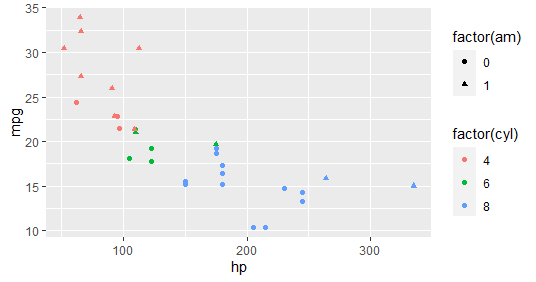• Geometric layer – Histogram plot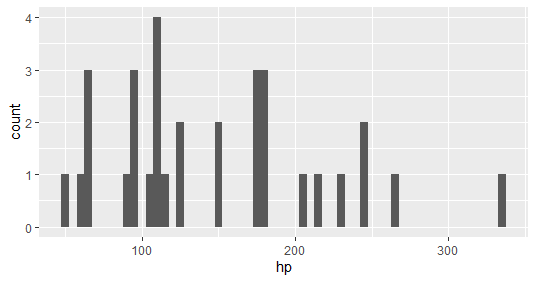• Facet layer – Separate rows according to transmission type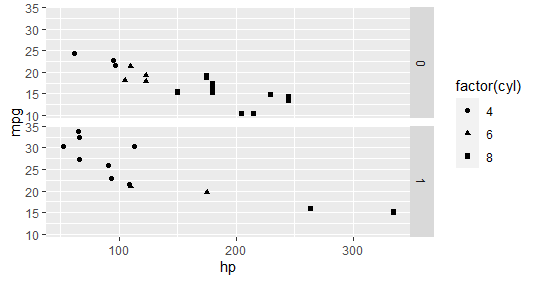• Facet layer – Separate columns according to cylinders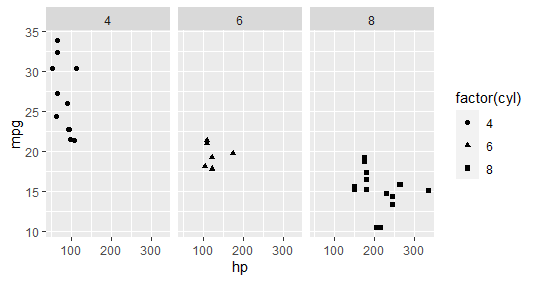• Statistics Layer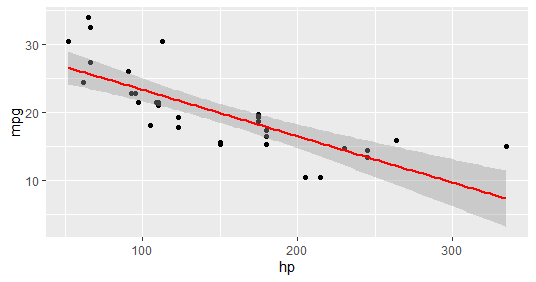• Coordinates layer: Control plot dimensions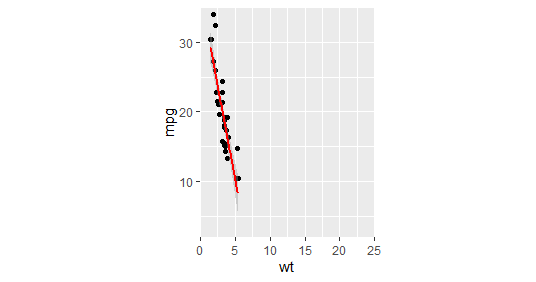• Coord_cartesian() to proper zoom in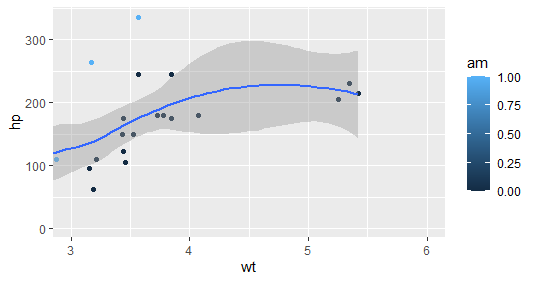• Theme layer – element_rect() function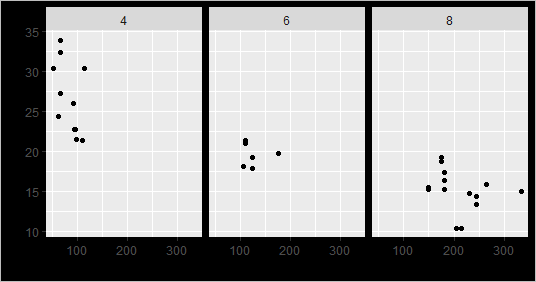• Theme layer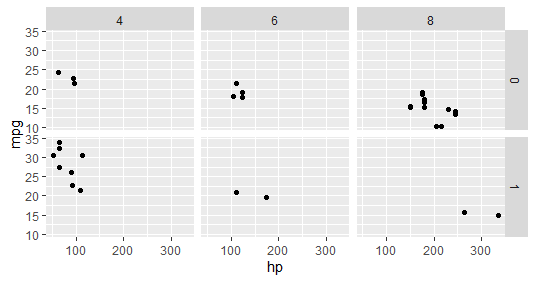ggplot2 provides various types of visualizations. More parameters can be used included in the package as the package gives greater control over the visualizations of data. Many packages can integrate with the ggplot2 package to make the visualizations interactive and animated.

My Personal Notes arrow_drop_up